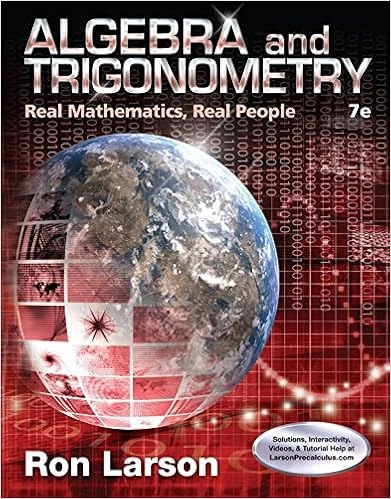• 4

This preview shows page 1 - 2 out of 4 pages.

##### We have textbook solutions for you!
The document you are viewing contains questions related to this textbook.The document you are viewing contains questions related to this textbook.
Chapter 7 / Exercise 48
Algebra and Trigonometry: Real Mathematics, Real People
LarsonExpert Verified
Group 2 Homework 1 MULTIPLE CHOICE. Choose the one alternative that best completes the statement or answers the question. Find the magnitude. 1) Let u = 2, - 2 and v = 4, 5 . Find the magnitude (length) of the vector: - 2 u - v . 1) A) 65 B) 59 C) 65 D) 59
Find the acute angle, in degrees, between the lines. 2) y = 3 x + 16 and y = - 3 x + 2 2)
Express the vector in the form v = v 1 i + v 2 j + v 3 3) P 1 P 2 if P 1 is the point (4, 4, - 4) and P 2 is the point (6, 1, - 8) A) v = - 2 i + 3 j + 4 k B) v = - 2 i - 3 j + 4 k C) v = 2 i - 3 j - 4 k D) v = - 2 i + 3 j - 4 k Find the distance between points P 1 and P 2 . 4) P 1 (5, - 9, 10) and P 2 (15, 1, 15) 4) k. 3)
Express the vector as a product of its length and direction. 5) 6 i + 12 j + 12 k 5) k
Solve the problem. 6) Find a vector of magnitude 9 in the direction opposite to the direction of v = 1 2.5 i + 1 2.5 j - 1 2.5 k . 6) A) 9 1 2.5 i + 1 2.5 j - 1 2.5 k B) 7.5 3 1 2.5 i + 1 2.5 j - 1 2.5 C) 7.5 3 - 1 2.5 i - 1 2.5 j + 1 2.5 k D) 7.5 - 1 2.5 i - 1 2.5 j + 1 2.5 k k
7)
##### We have textbook solutions for you!
The document you are viewing contains questions related to this textbook.The document you are viewing contains questions related to this textbook.
Chapter 7 / Exercise 48
Algebra and Trigonometry: Real Mathematics, Real People
LarsonExpert Verified
•••